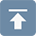# Steel structure budget calculation rules

## Steel structure budget calculation rules

• 06 Dec 2019
• steel structure

Steel Structure Budget Precautions

1. The materials must be distinguished,different steel materials have different prices (such as ordinary profile steel, Z / C steel, ordinary steel and angle steel, etc., the price should be calculated separately, should not be packed together)

2. Pay attention to whether the steel used in the quota is consistent with the material in the quota, and whether the content is consistent. If it is inconsistent, it should be adjusted.

3. It is specified that all steel structural members must be painted with fireproof coatings, and small components cannot be missed.

4. The connection way of steel structure nodes must be specified, then the construction process can not be missed.

The main reasons which influence the steel structure cost

1. The conservative degree of design.

2. Market material prices.

3. The level of the construction team (directly affects the loss and speed of production, transportation, installation).

4. Fireproof coatings are not a small expense.

Steel structure engineering quantity calculation rule

No. Item Components Name Diagram Measurement unit Engineering Amount Calculation Rules Notes
Light steel 1 Embedded part Pre-embedded anchor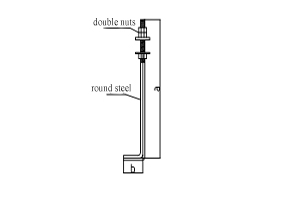set Calculated separately according to specifications and length
Budget quotation: classified by specifications and Calculated quotation by number of sets
Internal settlement: calculated in tonnage = length (a + b) * theoretical weight of the specification, nuts, pads need to be calculated separately
(round steel theoretical weight = 0.00617 * d2)
The total number: the number of anchor bolts (refer to the anchor bolt layout)
Embedded parts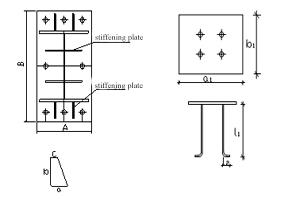T 1. Steel column embedded parts:
(1)Column foot board: A*B* theoretical weight of this specification
(2)Stiffening plate: (a+c)*b/2* theoretical weight of the specification
2. The door frame column embedded parts:
(1)pre-embedded board: a1*b1* theoretical weight of this specification
(2)screw rod: (L1 + L2) * theoretical weight of the specification
(Steel plate theoretical weight = 7.85*t)
(round steel theoretical weight = 0.00617 * d2)
The engineering quantity of the column foot board and stiffening plate of the steel column is incorporated into the steel column engineering quantity, and the embedded parts, such as door frame column is separately.
Light steel 2 Steel Column Parts Steel column
(H types)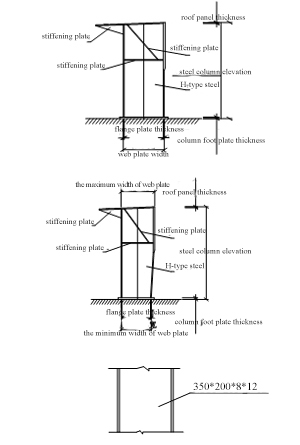T (1) Steel column (uniform section):
Flange plate = (steel column top elevation - column bottom plate bottom elevation - column foot plate thickness - top gusset plate thickness) * flange plate width * theoretical weight of the flange plate
Web plate = (steel column top elevation - column bottom plate bottom elevation - column foot plate thickness - top gusset plate thickness) * (this web plate section height - two flange plates thickness) * theoretical weight of the web plate
(2) Steel column (variable section):The calculation method of the flange plate is the same as that of the uniform-section column flange plate.
Web plate= (steel column top elevation - column bottom plate bottom elevation - column foot plate thickness - top gusset plate thickness) * (average of the maximum section height and minimum section height of the web plate - two flange plates thickness) * Theoretical weight of web plate
(3) steel column (uniform section)
Actual height of the steel column * theoretical weight of this model
[Theoretical weight calculation method: flange plate width * theoretical weight of the flange plate * 2 (web plate section height - two flange plates thickness) * theoretical weight of the web plate]
(theoretical weight of steel plate＝7.85*t)
1. When calculating the steel column engineering quantity ,the engineering quantity of the relevant gusset plate should be calculated according to the detailed drawing of the nodes (for example, the shape of the gusset plate is irregular or the polygonal steel plate is calculated with the minimum external rectangular area * the theoretical weight of this specification) The amount of engineering is incorporated into the steel column.
2. The project has a crane beam, and the beef leg (H-beam) is welded on the steel column. The calculation method is the same as the calculation method of the steel beam, and the engineering quantity is incorporated into the steel column engineering quantity.
3. The total weight: unit weight of the same steel column * numbers * number of points (refer to the steel frame layout)
Light steel 3 Steel Beam Parts Steel Beam
(H types)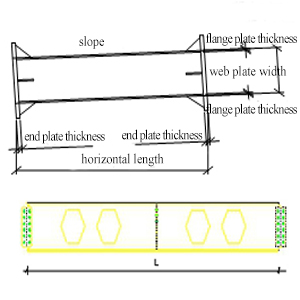T (1) Steel beams (the roof has a slope):
Flange plate = (the actual length of the beam - the thickness of the end gusset plate) * the width of the flange plate * the theoretical weight of the flange plate
Web plate = (the actual length of the beam - the thickness of the end gusset plate) * (the height of the web plate section - the thickness of the two flange plates) * the theoretical weight of the web plate
(2) Steel beam (honeycomb beam):
Actual length of the beam * theoretical weight of this model
[Theoretical weight calculation method: flange plate width * theoretical weight of the flange plate * 2 (web section height - two flange plates thickness) * theoretical weight of the web plate] (need to subtract hole area in which s hole area occupied by 0.3m2 or more on the web plate)
(theoretical weight of steel plate＝7.85*t)
1. Actual length = [(horizontal length * slope) 2 + horizontal length 2] arithmetic square root, slope is the ratio of vertical height to horizontal width
2. when calculating the engineering quantity of the steel beam,the engineering quantity of relevant gusset plate will be calculated according to the detail of the detailed drawings of node (for example, the shape of the gusset plate is irregular or the polygonal steel plate is calculated with the minimum external rectangular area * the theoretical weight of the specification) The amount of engineering quantity is incorporated into the steel beam.
3.If the steel beam is hot-rolled H-shaped steel, high-frequency H-shaped steel, T-shaped steel, etc. They will be calculated according to the actual length * theoretical weight of the model [high-frequency H-shaped steel theoretical weight calculation method: flange plate width * theoretical weight of flange plate 2 + (web plate section height - two flange plate thickness) * theoretical weight of the web plate; hot rolled H-beam is the finished products, so the theoretical weight please check the weight table]
Light steel 4 Purlin and angle brace purlin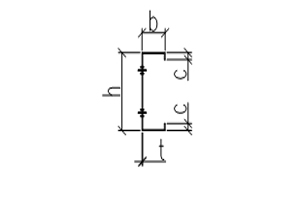T Length * theoretical weight of the specification
[Note: If the purlin is C-type steel, its theoretical weight (kg/m) = (h+2b+2c- 4t)*t*0.00785]
1. Calculate the engineering quantity of joint plate (steel plate area * theoretical weight of this specification) according to the detailed drawing of the purlins node, and the engineering quantity will be included in the roof purlin.
2. the total weight: the weight of a single purlin * the number of purlins(refer to the purlin layout)
Light steel 5 Angle brace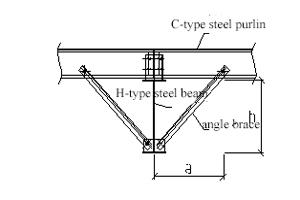T Oblique length * theoretical weight of this specification
1. If the deep drawing has not yet been finished, calculate the engineering quantity according to the construction drawing (without node): [As shown in the figure: Angle brace length = (the height of the steel beam h2+ the horizontal distance a2 of angle brace from the end of the steel beam connected to the end of the rafter)The square root of the arithmetic +50mm]
2. If the deep drawing has been finished, the length of the angle brace is calculated according to the length in the deep drawing.
[Theoretical weight of angle steel (kg/m) = 0.00795* t* (2 b-t) or you can check the hardware manual]
1. Calculate the engineering quantity of joint plate (steel plate area * theoretical weight of this specification) according to the detailed drawing of the joints, and the engineering quantity will be included in the roof purlin.
2. The total weight: the weight of a single angle brace * the number of angle brace(refer to the purlin layout)
Light steel 6 Roof small Pieces Brace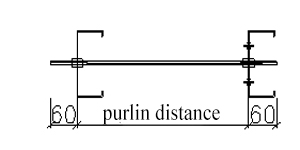T (purlin distance+ add 60mm at both ends) * Theoretical weight of this specification
〔theoretical weight of round steel(kg/m)＝0.00617*d2〕
1. If it is near the gutter side, the length of the brace = the distance between the purlins +one end 60mm
2. the total weight: weight of single bar* number of bars (refer to the layout of purlin)
Light steel 7 Horizontal support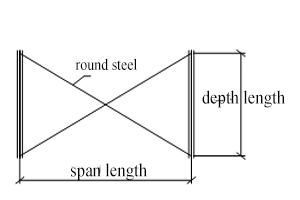T Oblique length * theoretical weight of this specification
[As shown in the figure: oblique length = (span length a2 + depth length b2)] arithmetic square root
[The theoretical weight of round steel (kg/m) = 0.00617*d2]
[Theoretical weight of angle steel (kg/m) = 0.00795* t* (2 b-t) or you can check the hardware manual]
1. The engineering amount of angle steel at the four ends of the horizontal support is incorporated into the horizontal support calculation
2. Total weight:weight of single horizontal support * horizontal support numbers (refer to the roof structure layout)
Light Steel 8 Round tube pressure rod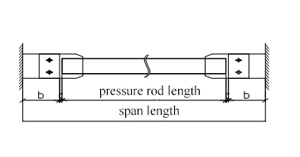T Actual length * theoretical weight of this specification
[As shown in the figure: length = (axis span length a-2b-2c)]
The theoretical weight of round steel (kg/m) = 0.02466 * wall thickness * (steel pipe diameter - wall thickness)
1. Calculate the gusset plate engineering quantity (steel plate area * the theoretical weight of the specification) according to the gusset detailed drawing, and the engineering quantity is incorporated into the steel beam.
2. Total weight: the weight of a single round pipe pressing rod * the number of round pipe pressing rods (refer to the roof structure layout)
Light Steel 9 Steel plate gutter
(stainless steel gutter)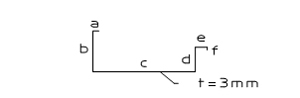T Actual length * spread width * theoretical weight of the specification
(Theoretical weight of steel plate = 7.85 * t)
1. The head plate at the two ends of the gutter is calculated based on the steel plate area * theoretical weight, and the engineering amount is incorporated into the gutter.
2. Total weight: the weight of a single gutter * the number of gutters (refer to the roof architectural drawing)
Light Steel 10 Gable wall angle steel T Gable wall oblique length * Theoretical weight of this specification Total weight: single-sided gable wall angle steel weight * two gable walls
Light Steel 11 Wall Panel and Small Components Wall beam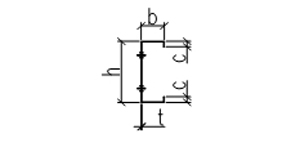T Length * Theoretical weight of the specification
[Note: If the wall beam is C-section steel, its theoretical weight (kg / m) = (h + 2b + 2c- 4t) * t * 0.00785]
1. Calculate the gusset plate engineering quantity (plate area * theoretical weight of the specification) according to the detailed drawings of the wall beam joints, and the engineering quantity is incorporated into the steel column.
2. If the wall beam meets the door and window opening, the length should be deducted from the size of the door and window opening. The farthest end wall beam should protrudes some distance from the steel column (marked in the drawing).
3. Total weight: the weight of a single wall beam * the number of wall beams (refer to the wall beam layout)
Light Steel 12 Vertical support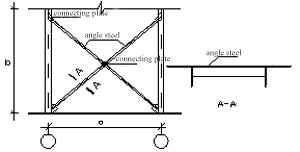T Oblique length * theoretical weight of the specification
1. If the detailed drawing has not yet been finished, the engineering quantity can only be calculated according to the construction drawing: as shown in the figure: oblique length = [(vertical support height b-distance between the gusset plates at both ends) 2+ (distance between two steel columns a- Distance between the two end gussets) 2] Arithmetic square root] + 50mm
2. If the detailed drawing has been finished, the length will be calculated according to the detailed drawing.
The vertical support is a stepped angle steel support, and the angle steel connecting the two angle steels is calculated according to the distance between the two angle steels * theoretical weight * number of steps
1. Total weight: weight of single vertical support* number of vertical support (refer to wall beam layout)
2. Calculate the gusset plate engineering quantity (steel plate area * theoretical weight of the specification) according to the detailed drawing of support joints, and the engineering quantity is incorporated in the vertical support.
Light Steel 13 Small Components Door frame
Window Frame
--- T the length of the door beam, door frame and window frame * the theoretical weight of the specification 1. Calculate the embedded parts and gusset plate of the door frame and window frame according to the joints drawing (steel plate area * theoretical weight of the specification)
2. Total weight: single weight * number (refer to wall beam layout)
Light Steel 14 Wall Brace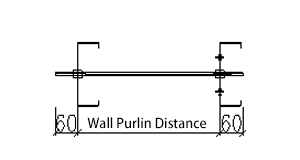T (Wall beam distance + 60mm at each end) * Theoretical weight of this specification
(Theoretical weight of round steel (kg / m) = 0.00617 * d2)
1. If it is near the gutter side, the length of the brace = the distance between the wall purlins + 60mm
2. the total weight: weight of single brace* number of braces (refer to the layout of wall purlin)
Light Steel 15 Crane Crane beam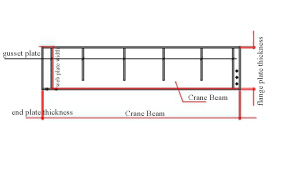T The calculation method is the same as that of the steel beam:
Flange plate = (length of crane beam-end gusset plate thickness) * flange plate width * theoretical weight of flange plate
Web plate = (length of crane beam-thickness of end gusset plate) * (height of web plate section -thickness of two flange plates) * theoretical weight of web plate
1. When calculating the crane beam quantity, calculate the relevant gusset plate engineering quantity according to the detailed drawings of the joints (if the gusset plate shape is irregular or polygonal, steel plate is calculated with its minimum external rectangular area * the theoretical weight of the specification) The engineering amount is incorporated into the crane beam.
2. Total weight: weight of single crane beam * number of crane beams
Light Steel 16 System Part Braking System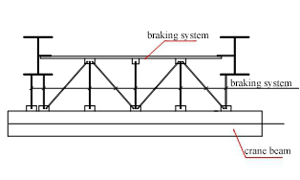T The actual length of the components that form the braking system * The theoretical weight of the specification
(Brake system is generally angle steel, channel steel)
[Theoretical weight of angle steel (kg / m) = 0.00795 * t * (2 b-t) or you can check the hardware manual]
[Theoretical weight of channel steel (kg / m) = (h + 2b- 2t) * t * 0.00785]
1. When calculating the braking system engineering quantity, calculate the relevant gusset plate engineering quantity according to the detailed drawings of the joints (if the gusset plate shape is irregular or polygonal, steel plate is calculated with its minimum external rectangular area * the theoretical weight of the specification)
2. Total weight: weight of single braking system * number of braking system(The number of braking systems is equal to the number of segments of the crane beam)
Light Steel 17 Car stopT The calculation method is the same as that of the steel column:
Flange plate = (car stop height-bottom plate thickness) * flange plate width * theoretical weight of flange plate
Web plate = (car stop height- bottom plate thickness) * (height of this web section-thickness of two flange plates) * theoretical weight of web plate
(If you actually encounter different car stop structures, the calculation will be based on actual calculations)
1. When calculating the car stop engineering quantity, calculate the relevant gusset plate engineering quantity according to the detailed drawings of the joints (if the gusset plate shape is irregular or polygonal, steel plate is calculated with its minimum external rectangular area * the theoretical weight of the specification)
2. Total weight: weight of single car stop * number of car stop(generally there are one car stop in each end of crane beam )
Light Steel 18 Floor Beam Part Main beam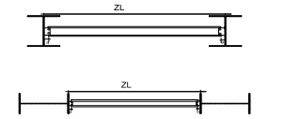T The calculation method is the same as that of the steel beam:
Flange plate = (beam length-distance between end gusset plate and beam a * 2) * flange plate width * theoretical weight of flange plate
Web plate= (Beam length-distance from end gusset plate to beam a * 2) * (web plate section height-thickness of two flange plates) * theoretical weight of web plate
[Beam length = distance between the centerline of the column web plate-web plate thickness of the column (or flange plate thickness)]
1. When calculating the engineering amount of floor main beams, calculate the relevant gusset plate engineering amount in accordance with the detailed joints drawing and incorporate the amount into the main beam.
2. Total weight: the weight of a single main beam * the number of main beams (refer to the floor beam layout)
Light Steel 19 Secondary beam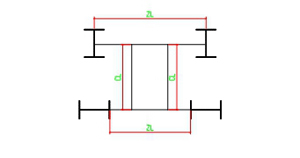T The calculation method is the same as that of the steel beam:
Flange plate = (beam length-distance between end gusset plate and beam a * 2) * flange plate width * theoretical weight of flange plate
Web plate= (Beam length-distance from end gusset plate to beam a * 2) * (web plate section height-thickness of two flange plates) * theoretical weight of web plate
[Beam length = distance between the centerline of the main beam web plate-web plate thickness of the main beam )
1. When calculating the engineering amount of floor secondary beams, calculate the relevant gusset plate engineering amount in accordance with the detailed joints drawing and incorporate the amount into the secondary beam.
2. Total weight: the weight of a single secondary beam * the number of secondary beams (refer to the floor beam layout)
Light Steel 20 Clerestory Clerestory frame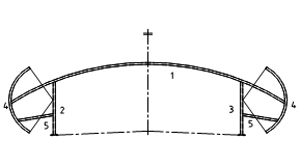T Component ①, ②, ③, ④, ⑤ total spread length * theoretical weight of the specification 1. When calculating the engineering quantity of clerestory frame, calculate the relevant gusset plate engineering amount according to the detailed joints drawing and incorporate into the gusset plate separately.
2. Total weight: the weight of a single clerestory frame * the number of clerestory frame (refer to the layout of the clerestory)
Light Steel 21 Clerestory beam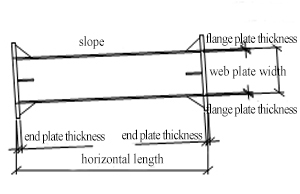T The calculation method is the same as that of the steel beam:
Flange plate = (beam length-end gusset plate thickness) * flange plate width * theoretical weight of flange plate
Web plate = (beam length-end gusset plate thickness) * (web plate section height-thickness of two flange plates) * theoretical weight of web plate
1. When calculating the engineering quantity of clerestory beam, calculate the relevant gusset plate engineering amount according to the detailed joints drawing and incorporate into the gusset beam.
2. Total weight: the weight of a single clerestory beam * the number of clerestory beam (refer to the layout of the clerestory)
Light Steel 22 Notes: Clerestory small components(ie. The calculation rules of purlins and wall beams are the same as those small components of the roof and wall)
Light Steel 23 Canopy Cantilever beam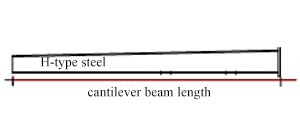T The calculation method is the same as that of the steel beam:
Flange plate = (cantilever beam length-end gusset plate thickness) * flange plate width * theoretical weight of flange plate
Web plate = (cantilever beam length-end gusset plate thickness) * (web plate section height-thickness of two flange plates) * theoretical weight of web plate
(Such as the calculation rules of variable section beams is same with that of steel frame beams)
1. When calculating the engineering quantity of cantilever beam, calculate the relevant gusset plate engineering amount according to the detailed joints drawing and incorporate into the cantilever beam.
2. Total weight: the weight of a single cantilever beam * the number of cantilever beam (refer to the canopy structural drawing)
Light Steel 24 Steel gutter
(Stainless steel gutter)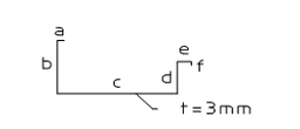T Actual length * spread width * theoretical weight of the specification
(Theoretical weight of steel plate = 7.85 * t)
1. The head plate at the two ends of the gutter is calculated according to the steel plate area * theoretical weight, and the engineering amount is incorporated into the steel gutter(stainless steel gutter).
2. Total weight: the weight of a single gutter * the number of gutters (refer to the canopy structural drawing)
Light Steel 25 Self-folding steel plate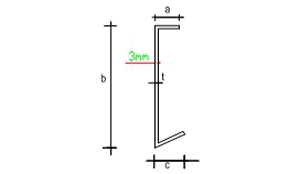T Spread width of steel plate * canopy length * Theoretical weight of this specification
Theoretical weight = (a + a + c-2t) * t * 7.85
Light Steel 26 Angle steel --- T Length * Theoretical weight of this specification The length of this angle steel is equal to the spread width of the canopy.
Light Steel 27 Notes: canopy small components (ie: The calculation method of purlins and brace is same to that of small components of roof)
Light Steel 28 Steel Stair Part Stair column --- T The calculation method is the same as that of the steel column:
Flange plate = (Stair column height-column foot plate thickness-Roof plate thickness) * Flange plate width * theoretical weight of flange plate
Web plate= (Stair Column Height-column foot Thickness-Roof Thickness) * (Height of This Web plate section-Thickness of Two Flange Plates) * Theoretical Weight of web plate
1. When calculating the engineering amount of stair columns, calculate the engineering amount of related gusset plates according to the detailed joints drawings and incorporate the engineering amount into the stair columns.
2. Total weight: the weight of a single stair column * the number of stair columns
Light Steel 29 Stair beam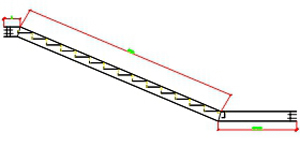T The calculation method is the same as that of the steel beam:
Flange plate = (total length of stair beam-end gusset plate thickness) * flange plate width * plate thickness * theoretical weight of flange plate
Web plate = (total length of stair beam-thickness of end gusset plate) * (height of web section-thickness of two flange plates) * theoretical weight of web plate
(As shown in the figure: the total length of the stair beam = the length of the inclined stair beam a + b + the length of the resting platform c)
1. When calculating the engineering amount of stair beams, calculate the engineering amount of related gusset plates according to the detailed joints drawings and incorporate the engineering amount into the stair beams.
2. Total weight: the weight of a single stair beam * the number of stair beams
Light Steel 30 Step board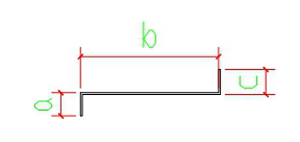T Stepping board spread width * actual net width * theoretical weight of this specification
(As shown in the figure: stepped board spread width = a + b + c)
Total weight: weight of single step board * number of step boards
Light Steel 31 handrail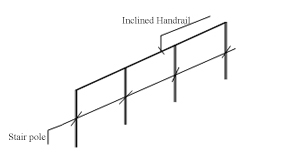T Extension meter * The theoretical weight of this specification
(As shown in the figure: the meters of the handrail include the meters of the vertical bar and the meters of the inclined railing)
If the material of the handrail and railing is stainless steel, it is calculated by extension meter.
Light Steel 32 Hanging platform
Pperating platform
It is a platform composed of columns, beams, plates, diagonal braces, etc. and some have steel stairs handrail and platform railings etc surrounding components T The columns, beams, and plates of the platform (calculated according to the laying area shown in the figure without deducting the hole area less than 0.3 m2), the diagonal braces are calculated according to the corresponding component calculation rules and are incorporated into the steel platform. The stair and handrail are calculated according to corresponding calculation rules and incorporated into staircase quantities
Light Steel 33 Maintenance ladder --- T If the detailed diagram of the maintenance ladder is not shown in the drawing. Please refer to the maintenance ladder atlas. Calculation method of the engineering quantity: length * the theoretical weight of this specification(calculate the engineering amount of related gusset plates according to the detailed drawings and incorporate the engineering amount into the maintenance ladder)
No. Item Components Name Diagram Unit Engineering Amount Calculation Rules Notes
Heavy Steel 1 Roof Frame Steel Roof Frame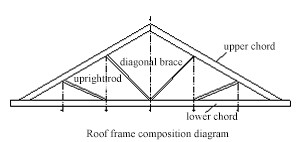T

The actual net length of the upper and lower chords, upright rods and diagonal braces constituting the steel roof frame * the theoretical weight of the corresponding specifications 1. When calculating the roof frame engineering quantity, calculate the relevant gusset plate engineering quantity according to the detailed drawings of the joints (if the gusset plate shape is irregular or polygonal, steel plate is calculated with its minimum external rectangular area * the theoretical weight of the specification) and incorporated the engineering quantity into steel roof frame.
2. Total weight: weight of single roof frame * number of same units
Heavy Steel 2 Steel Column Lattice column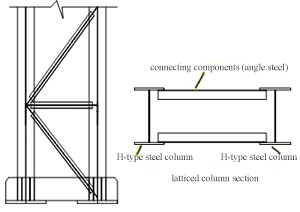T 1.Calculate the weight of the next two steel columns according to the calculation rules of Light Steel steel columns.
2.The actual net length between the two steel web plates*the theoretical weight of the corresponding specifications to calculate the weight of steel connecting components(normally the connecting components are angle steel)
1. When calculating the lattice column engineering quantity, calculate the relevant gusset plate engineering quantity according to the detailed drawings of the joints (if the gusset plate shape is irregular or polygonal, steel plate is calculated with its minimum external rectangular area * the theoretical weight of the specification) and incorporated the engineering quantity into lattice column.
2. When calculating the connecting components, the second line should be mentioned.
Heavy Steel 3 Notes: Solid web steel columns, steel supports (round pipe pressure rod, brace, corner braces, horizontal supports, etc.), crane beams and brake beams, etc. refer to the calculation rules for Light Steel projects
No. Item Components Name Diagram Unit Engineering Amount Calculation Rules Notes
High level 1 Steel Column Part Box type column (polygonal column, special-shaped column)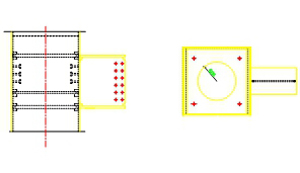T The first section of steel column: (steel column height-column foot thickness) * (side length deduct the overlap thickness * the theoretical weight of this specification); if it is n-sided shape, the total weight is the sum of the weights of n sides
Steel columns with more than one section: the actual height of the steel column * (side length deducts the overlap thickness * the theoretical weight of the specification); if it is n-sided, the total weight is the sum of the weights of n sides
(Theoretical weight of steel plate = 7.85 * t)
1. The elevation of each section of the steel column refers to the steel column table (or steel column elevation)
2. Gusset plates such as partitions and plywood in box-shaped columns are calculated by referring to the "column-to-beam connection node", and the engineering quantity incorporated into the column.
3. If the hole area is less than 0.3m2, the hole area is not deducted; if it is more than 0.3m2, the hole area should be deducted
4.The number of bolts sets on the column is calculated separately according to the number of sets
Cross column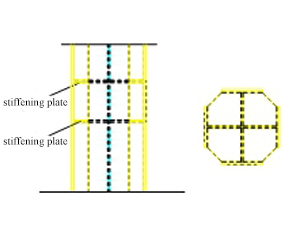T The first section of steel column: (steel column height-column foot plate thickness) * (weight per meter of one H-shaped steel column forming the cross column + weight per meter of two T-shaped steels forming the cross column)
Steel columns with more than one section: actual height of the steel columns * (weight per meter of one H-shaped steel column forming the cross column + weight per meter of two T-shaped steels forming the cross column)
1. The elevation of each section of the steel column refers to the steel column table (or the steel column elevation)
2. The gusset plates such as the partitions of the cross column are calculated by referring to the "connection joints between the column and the beam", and the engineering quantity is incorporated into the column
Round pipe column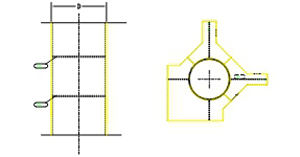T he first section of steel column: (steel column height-column foot plate thickness) * the theoretical weight of the round tube of this specification
Steel columns with more than one section: the actual height of the steel column * the theoretical weight of the round pipe of this specification
Round pipe theoretical weight (kg / m) = 0.02466 * wall thickness * (steel pipe diameter-wall thickness)
1. The elevation of each section of the steel column refers to the steel column table (or the steel column elevation)
2. The gusset plates such as partitions of round pipe columns are calculated with reference to the "column-to-beam connection joints", and the engineering quantities are incorporated into the columns.
3. Circumferential ring plates of round pipe columns are calculated according to the smallest circumscribed rectangle. If the hole area is within 0.3m2, the hole area is not deducted; if it is above 0.3m2, the hole area should be deducted.
High level 2 Level Beam Main beam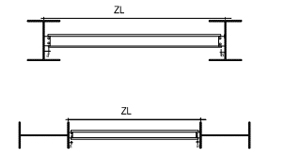T The calculation method is the same as that of the steel beam:
Flange plate = (beam length-distance between end gusset plate and beam a * 2) * flange plate width * theoretical weight of flange plate
Web plate= (Beam length-distance from end gusset plate to beam a * 2) * (web plate section height-thickness of two flange plates) * theoretical weight of web plates
[Beam length = distance from the centerline of the column web plate-web plate thickness of the column (or flange plate thickness)]
1. When calculating the engineering amount of level main beams, calculate the relevant gusset plate engineering amount according to the detailed joints drawing and incorporate the amount into the main beam.
2. Total weight: the weight of a single main beam * the number of main beams (refer to the level beam layout)
High level 3 Secondary Beam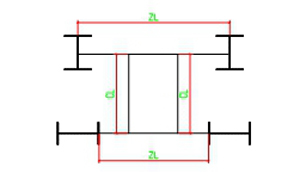T The calculation method is the same as that of the steel beam:
Flange plate = (beam length-distance between end gusset plate and beam * 2) * flange plate width * theoretical weight of flange plate
Web plate= (Beam length-distance from end gusset plate to beam * 2) * (web plate section height-thickness of two flange plates) * theoretical weight of web plates
[Beam length = distance from the centerline of the main beam web plate-web plate thickness of the main beam web plate
1. When calculating the engineering amount of level secondary beams, calculate the relevant gusset plate engineering amount according to the detailed joints drawing and incorporate the amount into the secondary beam.
2. Total weight: the weight of a single secondary beam * the number of secondary beams (refer to the level beam layout)
High level 4 Steel beam Box-type beam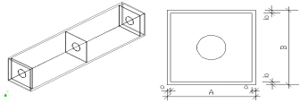T Box-type beam: actual net beam length * theoretical weight of the specification
Box-type beam theoretical weight: [B * thickness * 7.85kg / m3 * 2 + (A-2a) * thickness * 7.85kg / m3 * 2]
1. The gusset plates such as partitions plate, plywood, etc. in the box-type beam are calculated by referring to the detailed joints drawing, and the engineering amount is incorporated into the calculation of the beam.
2. If the hole area of gusset plate such as a single stiffener plate or partition, plywood and other n is less than 0.3m2,don’t need to deduct the hole area; if it is more than 0.3m2, the hole area should be deducted.
3. Total weight: the weight of a single box-type beam * the number (refer to the layout of the steel beam)
High level 5 Special-shaped Beam Steel beams with irregular cross-sections size, such as curved beams, etc. T The corresponding calculation rules of the members constituting the special-shaped beam When calculating the engineering amount of special-shaped beams, calculate the engineering quantity of the relevant gusset plate according to the detailed joints drawings (if the shape of the gusset plate is irregular or the polygonal steel plate, calculate it by its smallest external rectangular area * plate thickness * 7.85kg / m3).
High level 6 Notes: The engineering amount calculation method of H-type steel columns, column bracing (such as vertical supports), steel beam supports (such as horizontal supports, angle brace, round pipe pressure rods, etc.), steel stairs, and steel railings refer to the calculation rules of light steel project
No. Item Components Name Diagram Unit Engineering quantity calculation rule Notes
Space 1 Truss Part Chord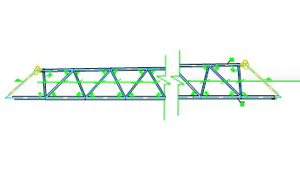The above picture shows the round tube truss. If it is rectangular tubes and angle steel trusses, the calculation rules are the same as those for round tubes, but the theoretical weight is different.
T Chord length * Theoretical weight of round pipe of this specification 1. When calculating, calculate the relevant gusset plate engineering quantity according to the detailed joints drawing (if the gusset plate is irregular or the polygonal steel plate ,then calculated by its minimum circumscribed rectangular area * theoretical weight), and the engineering quantity is incorporated into the gusset plate.
2.The connecting ball at the end is calculated based on the volume * theoretical weight
Space 2 Web rod T Length between two chords * Theoretical weight of the round tube of this specification 1. When calculating, calculate the relevant gusset plate engineering quantity according to the detailed joints drawing (if the gusset plate is irregular or the polygonal steel plate ,then calculated by its minimum circumscribed rectangular area * theoretical weight), and the engineering quantity is incorporated into the gusset plate.
2.The web rod has an upper web rod that connects the two upper chords and a lower web rod that connects the upper and lower chords.
Space 3 Net frame part Net frame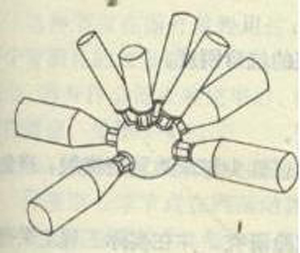T The actual net length of the connecting rod between the two net frame balls * the theoretical weight of the corresponding specification The net frame ball is calculated based on the volume of ball * theoretical weight
Space 4 Maintaining roadway The roadway is used for house maintenance, and is generally composed of beams, steel plates, supports and other components T The beams and plates of the maintaining roadway (calculated according to the laying area shown in the figure without deducting the hole area within 0.3m2), diagonal braces, railings, etc., calculate the weight according to the corresponding components calculation rules and incorporate them into the maintaining roadway Irregular or polygonal steel plates are calculated by the minimum circumscribed rectangular area * the thickness * the unit theoretical mass
Space 5 Inverted grid roof ceiling suspended from above by a boom or the like T Calculate the engineering amount of each member that forms the inverted grid top in accordance with the corresponding calculation rules Irregular or polygonal steel plates are calculated by the minimum circumscribed rectangular area * the thickness * the unit theoretical mass
Space 6 Purlin hanger base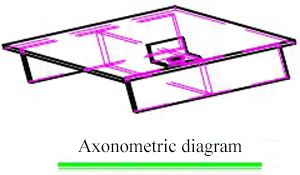Axonometric diagram
Connecting plate uses for upper chord of truss and purlin hanger
T Illustrated size of steel plate (calculated by area) * Unit theoretical weight Irregular or polygonal steel plates are calculated by the minimum circumscribed rectangular area * the thickness * the unit theoretical mass
Space 7 Notes: The engineering amount calculation of section steel purlin wall beams, steel supports (round pipe pressure rod, horizontal supports, brace, etc.) are according to the above Light Steel calculation rules.

Panel engineering amount calculation rules

No. Item Components Name Diagram Unit Engineering quantity calculation rule Notes
1 Roof panel Upper layer plate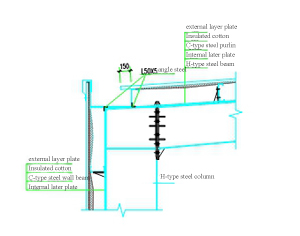m2 (steel frame span length+ length of wall beam protruding from steel column-width of steel plate gutter + length of roof panel extending from gutter 150mm + roof ridge upwards length 50mm) * roof slope coefficient * (total length of bay + length of wall beam extending from steel column) 1. If there is a skylight or clerestory,the area of skylight and clerestory should be deducted.
2. as for the opening of the fan and the opening of the contact should be open on-site,and the area should not be deducted.
3. In this diagram, the gutter is not an external extension. If the gutter is an external extension, the length of the roof panel = steel frame span length + the length of the roof panel extending the steel gutter 150mm + the roof ridge upwards length 50mm, that is, there is no need to subtract the steel plate gutter width, and the length of wall beam protruding the steel column will not increase.
2 Insulated cotton m2 (steel frame span length + length of wall beam protruding from steel column-width of steel gutter) * coefficient of roof slope * (total length of bay + length of wall beam protruding from steel column); if there is insulation cotton under the gutter, it does not deduct the gutter width, plus area on both sides of the gutter
3 Bottom layer plate m2 (steel frame span length + length of steel column gutter protruding from steel column) * coefficient of roof slope * (total length of bay + length of steel beam protruding from steel column)
4 Wall Panel External layer plate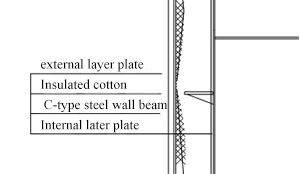m2 (Height of the wall-height of the brick wall + height of the part protruding into the brick wall) * (total length of the bay+ length of the steel beam protruding from the steel column)-door and window opening area For example, the hole to be opened in the contract list is an on-site opening, and the area should not be deducted.
5 Insulated cotton m2 (Height of the wall-height of the brick wall) * (total length of the bay + length of the steel beam protruding from the steel column)-area of the door and window opening For example, the hole to be opened in the contract list is an on-site opening, and the area should not be deducted.
6 Internal later plate m2 (The elevation of the bottom of the steel plate gutter-the elevation of the floor surface-the height of the brick wall) * the total length of the bay-the area of the door and window openings For example, the hole to be opened in the contract list is an on-site opening, and the area should not be deducted.
7 Internal eave coverage plate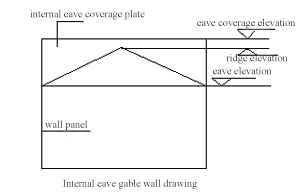m2 Front elevation: (Elevation of the eave coverage top-Eaves elevation) * Total length of the bay
Side Elevation (Symmetry): [(Elevation of Eaves coverage top-Eaves Elevation) + (Elevation of Eaves coverage top-Elevation of Roof ridge)] * Half of total bay length / 2 * Number of symmetrical blocks
Side elevation (asymmetry): [(elevation of eave coverage top-eaves elevation) + (elevation of eave coverage top-roof ridge elevation)] * the corresponding depth length / 2, the same method is used to calculate the other inner eave coverages
This calculation method is suitable for the situation that the eave coverage plate didn’t exceed the ridge, if on the contrary, calculate based on the diagram size.
8 Clerestory plate Side plate and top plate m2 Side plate: height of clerestory column * total length of clerestory + elevations on both sides
Roof panel: spread width of clerestory* Total Length of clerestory
9 Canopy plate Top layer plate m2 (Length of cantilever beam-wall beam width-steel plate gutter width + canopy upper plate protruding from gutter length 150mm) * canopy length
10 Insulated cotton m2 (Cantilever beam length-wall beam width) * canopy length
11 Bottom layer plate m2 (Cantilever beam length-wall beam width) * canopy length
12 Side plate m2 (steel gutter height+canopy cantilever beam height)*canopy spread length
13 Floor fascia Floor fascia m2 Level area-stair area-elevator shaft area-level area with an opening area above 3 m2
14 Downpipe system Φ200
Φ150
Φ100
Φ75
m The elevation from the bottom of the gutter to the design outdoor ground + 500mm, calculated in extended meters

Window and door engineering quantity calculation rules

No. Item Components Name Diagram Unit Engineering quantity calculation rule Notes
1 Window and Door Part window Aluminum sliding
Aluminum fixed
PVC sliding window
PVC fixed
Shutter
m2 Actual design opening area(length*width)
2 door Sliding door m2 (design door opening height+100mm)*(design door opening width+100mm*2) The unit price of sliding door includes the slide rail part, but the engineering quantity of the side rail part don’t need to be calculated
3 Rolling shutter door m2 door opening height+400mm)*(door opening width+150mm*2) The rolling door is Aino rolling door, and the electric system is included in the unit price of the door.If it is steel plate rolling shutter door, you also need to calculate the number of electric systems, one for each door
4 Vertical hinged door m2 Actual design opening area(length*width)
5 Swing door m2 Actual design opening area(length*width)
6 Clerestory plate Capron m2 (Circular arc clerestory top plate spread width + 500MM * 2) * clerestory length
7 Skylight plate Capron m2 (Circular arc top plate spread width + 500MM * 2) * skylight panel length

Anti-corrosion , fire-proof engineering amount calculation rules

No. Item Components Name Diagram Unit Engineering quantity calculation rule Notes
1 Anti-corrosion
Fire-proof
Engineering amount calculation rules
H-type steel H-type steel
I-type steel
m2 [(Upper flange plate width + lower flange plate width) * 2 + H-type steel height * 2] * component length If the H-type steel is used as the level beam, the surface of the upper flange plate does not need to be treated with anti-corrosion and fire protection, so the area don’t need to paint area should be deducted.
2 Closed components Box-type, buckle-type,trapezoid, rhombus, round tube, etc. m2 Perimeter of component section * component length
3 Steel plate m2 The steel plate area is calculated according to the size shown * 2 If if there is only one side of the steel plate treated by anti-corrosion and fire protection, the area is calculated according to the size shown